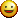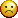###Author Topic: help with adding/subtracting/multiplying arrays in C++  (Read 2614 times)

0 Members and 1 Guest are viewing this topic.

#### Dreyth

• Hero Member
•• Posts: 2777
• Respect: +823##### help with adding/subtracting/multiplying arrays in C++
« on: February 10, 2013, 02:37:30 am »
0
So in C++ you can add multiply and subtract big numbers, right? Well i've created a class called BigInteger in which i want to be able to add subtract and multiply big ass numbers, as big as 40 digits long!!!

but the thing is i dont know how to come up with the algorithms to add them together. basically, the user inputs something like

"23412098308945341234234 * 2342093809823434243"

which is two operands (the two numbers aka op1 and op2) and the operator (the mult sign). I've already done the operator overloading.

When the user inputs the two numbers (op1 and op2) i want them to be put into an array, with each element representing 1 digit.

now the tricky part is... how can i get the arrays to add subtract and multiply properly? remember sometimes there will be a carry digit.

any help?
I'm LAKERS from The Vertical Summit

#### entropy

• Hero Member
••• Posts: 1684
• b00m!
• Respect: +276##### Re: help with adding/subtracting/multiplying arrays in C++
« Reply #1 on: February 10, 2013, 05:57:02 am »
0
First thing is that (fixed size) arrays are kind of fiddly here because you can overflow the target array size by using a sequence of operations which require more storage than you've allocated. Be careful about that. That's why i'd consider using either vectors or lists. Then you can grow the sequence as you need to. Are you ok with sometimes overflowing? If not then you should def switch to vectors or lists or some other variable length data structure.

The other thing is, if you care about (storage) efficiency then you're wasting a lot of space. Each element in your array is say 4 bytes long, and you're only storing one digit there? Super wasteful dude. But if you don't care about efficiency that's cool. If you want to be super efficient consider using bit vectors where you can compactly store a given number as efficiently as possible. But the only catch is you will have to add a bit of overhead in unpacking and packing these bit vectors. That's one way to do it. Just be careful of signs if you admit negative integers. If you have to worry about negative numbers work that into your representation (use 1s complement or whatever you like to keep a sign bit).

Anyway finally to answer your question, start by writing an algo that does plain school boy type addition. Yes you have to worry about carry but only once you've hit the end of one of the arrays. Btw once you've got addition, substraction is easy, just add the negative. And if you are clever you can setup multiplication as a series of additions. Leave this as an exercise for the reader lol.

But yea post some code and we'll give you some hints .. have fun!Goals: Cutting to 6-8% bodyfat

#### Dreyth

• Hero Member
•• Posts: 2777
• Respect: +823##### Re: help with adding/subtracting/multiplying arrays in C++
« Reply #2 on: February 10, 2013, 11:14:57 am »
0
^^ Yea I knowIf i'm able to handle overflow i get a little bonus for this assignment. i have to use arrays for this assignment. i'd much rather use vectors. ill post code in a few hours, thanks a lot for the input!
I'm LAKERS from The Vertical Summit

#### Dreyth

• Hero Member
•• Posts: 2777
• Respect: +823##### Re: help with adding/subtracting/multiplying arrays in C++
« Reply #3 on: February 10, 2013, 12:56:20 pm »
0
Okay so here is the HugeInteger class i have made as a header file:

Code: [Select]
`#ifndef HUGEINTEGER_H#define HUGEINTEGER_Husing namespace std;class HugeInteger{friend ostream& operator << (ostream&, const hugeInteger&);friend istream& operator >> (istream&, hugeInteger&);public:// Constructors:hugeInteger();hugeInteger(int [], int);// I/O functions:hugeInteger input (char);hugeInteger print();// Adding and multiplying functions:hugeInteger add(const hugeInteger&);hugeInteger mult(const hugeInteger&);// Operator overloading:bool operator > (const hugeInteger&);bool operator < (const hugeInteger&);bool operator == (const hugeInteger&);bool operator != (const hugeInteger&);const hugeInteger operator + (const hugeInteger&);const hugeInteger operator * (const hugeInteger&);private:const static int maxSize = 40;int array[maxSize + 1];int size;};#endif`

Now I'm currently working on the source file for HugeInteger where the implementation resides:

Code: [Select]
`#include "HugeInteger.h"#include <iostream>#include <cstdlib>#include <iomanip>using namespace std;// Default constructor to make 40 element arrayvoid main(){HugeInteger::HugeInteger()for (int i = 0; i < maxSize; i++)array[i] = 0;}// Copy constructor{HugeInteger::hugeInteger(int arr[], int b): size(b){if (size > maxSize) // Make sure arraysize = maxSize; // holds <= 40 elementsfor (int i = 0; i < size; i++) // Copy arrayarray[i] = arr[i];}// Input function{hugeInteger HugeInteger::input (char h)char b;while ((b = cin.get()) !h){if (b >= '0' && <= '9'){ // if a digit is inputarray[size] = atoi(&b); // atoi converts}}// string to int// Ostream function overload{{ostream &operator<< (ostream& out, const hugeInteger &h){bool value(false);int count;for (int i = 0; i < a.maxSize; i++){if (h.array[i] !=0){value(true);count = i;break;}{output<< h.array[i];return output;}// Output function{{hugeInteger Hugeinteger::print(){bool value(false);int count;for (int i = 0; i < maxSize; i++){if (array[i] !=0){value(true);cout = i;break;}{cout << array[i];}// Overload + for adding{const hugeInteger HugeInteger::operator+(const HugeInteger &h)add(h);return(h);}// Overload * for multiplying{{const hugeInteger HugeInteger::operator* (const HugeInteger &h)constmulti(h);return(h);}}// Overload == for equality{bool hugeInteger::operator== (const HugeInteger &h)constfor (int i = 0; i < maxSize; i++){if(array[i] > h.array[i]return true;elsereturn false;}// Overload > for greater than{bool hugeInteger::operator> (const HugeInteger &h)const{for (int i = 0; i < maxSize; i++){if(array[i] > h.array[i]return true;elsereturn false;}// Overload < for less than{bool hugeInteger::operator< (const hugeInteger &h)const{for (int i = 0; i < maxSize; i++)if(array[i] < h.array[i])return true;elsereturn false:}`
I'm LAKERS from The Vertical Summit

#### Dreyth

• Hero Member
•• Posts: 2777
• Respect: +823##### Re: help with adding/subtracting/multiplying arrays in C++
« Reply #4 on: February 10, 2013, 12:57:13 pm »
0
And right now I need to figure out the three algorithms and then make a driver program and link all three files.
But first, the adding algorithm I've come up with that DOESN'T work yet for some reason:

Code: [Select]
`void add(int a[], int b[], int result[]){    int carry = 0;    int right_digit = 0;    int answer = 0;    for (int i = 4; i >= 0; --i){ answer = a[i] + b[i] + carry; right_digit = answer % 10;        cout << "answer == " << answer << endl;        if (right_digit >= 10) {     carry = (answer - right_digit) / 10;        }        else {     carry = 0; } result[i] = answer;    }}`
for some damn reason the carry isn't being added to the next element... help?

edit:

I've found a pseudocode for the algorithm, but i'm stuck on trying to translate it into C++:

« Last Edit: February 10, 2013, 04:13:50 pm by Dreyth »
I'm LAKERS from The Vertical Summit

#### entropy

• Hero Member
••• Posts: 1684
• b00m!
• Respect: +276##### Re: help with adding/subtracting/multiplying arrays in C++
« Reply #5 on: February 11, 2013, 01:35:37 am »
0
And right now I need to figure out the three algorithms and then make a driver program and link all three files.
But first, the adding algorithm I've come up with that DOESN'T work yet for some reason:

Code: [Select]
`void add(int a[], int b[], int result[]){    int carry = 0;    int right_digit = 0;    int answer = 0;    for (int i = 4; i >= 0; --i){ answer = a[i] + b[i] + carry; right_digit = answer % 10;        cout << "answer == " << answer << endl;        if (right_digit >= 10) {     carry = (answer - right_digit) / 10;        }        else {     carry = 0; } result[i] = answer;    }}`
for some damn reason the carry isn't being added to the next element... help?

Just had a glance thru your code and noticed a few things that might be off. The right digit check (right_digit >= 10) you've got is unnecessary because % 10 will always return 0-9 anyway. So you're never going into that branch..
And since you're not going into that branch ... where are you going?
Goals: Cutting to 6-8% bodyfat

#### vag##### Re: help with adding/subtracting/multiplying arrays in C++
« Reply #6 on: February 11, 2013, 05:44:49 am »
0
Just had a glance thru your code and noticed a few things that might be off. The right digit check (right_digit >= 10) you've got is unnecessary because % 10 will always return 0-9 anyway. So you're never going into that branch..
And since you're not going into that branch ... where are you going?

Yup. Had a very fast look , seems like the problem is wrong interpretation of the decade system.

integer ABCDEFG = G*1 + F*10 + E*100 + D*1000 + C*10000 + B*100000 + A*1000000 , 0 <= A, B, C,... <= 9

Here's a fast pseudo ( not so pseudo, its C but not watching after syntax, doing declarations, mallocs etc ) of the sum code, pulled it right off my ass as im typing:
Code: [Select]
`array1[ASDFG...]array2[ZYZ...]for( i = max ( array1 length - 1 , array2 length - 1 ) ; i >= 0 ) ; i-- ){  excess = 0;  if( i > array1 length-1 ) val1 = 0;  else val1 = array1[i];  if( i > array2 length-1 ) val2 = 0;  else val2 = array2[i];  sum_val = val1+val2+excess;  if( sum_val >= 10 ){    sum_val -= 10;    excess = 1;  }  sum_array[i] = sum_val;}`
Now if there is excess coming out of the block it means the final array has 1 more digit, which can olny be 1, you must realloc the sumarray and prepend the value 1 in position 0 of the sum array. If excess == 0 coming out of the block no modifications are needed. In the former case, you may either malloc 1 extra place initially or realloc it in the end if needed.
Ill leave both those tasks to you!

Hope it helps!

edit:
For substraction, excess is not needed.
For multiplication , excess is the modulus of the multiplication and 10, final array may be MUCH longer than the initials.
Code: [Select]
`excess = (array1[i]*array2[i])%10`
« Last Edit: February 11, 2013, 06:25:45 am by vag »
woot

#### Dreyth

• Hero Member
•• Posts: 2777
• Respect: +823##### Re: help with adding/subtracting/multiplying arrays in C++
« Reply #7 on: February 12, 2013, 01:41:27 pm »
0
just some BS my friend came up with for multiplication:

Code: [Select]
`// Multiplication algorithm resides herehugeInteger hugeInteger::multiply(const hugeInteger &r) {    hugeInteger a;    hugeInteger c;       for (int i = 0; i < maxSize; i++) a.array[i] = 0;    int stop = 0;    for (int j = maxSize - 1; j >= 0; j--){ do{     for (int k = j; array[k] >= 0; k--){ int temp1 = c.array[k]; c.array[k] = (temp1 + (array[k]*r.array[j])) % 10; if ((temp1 + (array[k]*r.array[j])) > 9){     c.array[k - 1] = (temp1 + (array[k]*r.array[j]))/10; }     }     stop = 1; } while (stop == 0); for (int q = maxSize - 1; q >= 0; q--){     int temp2 = a.array[q];     int n = j - 1;     a.array[n] = (temp2 + (array[q]*r.array[j - 1])) % 10;     if ((temp2 + (array[q]*r.array[j - 1])) > 9){ a.array[n - 1] = (temp2 + (array[q]*r.array[j - 1]))/10;     } }    }    c.add(a);    return(c);}`
I'm LAKERS from The Vertical Summit